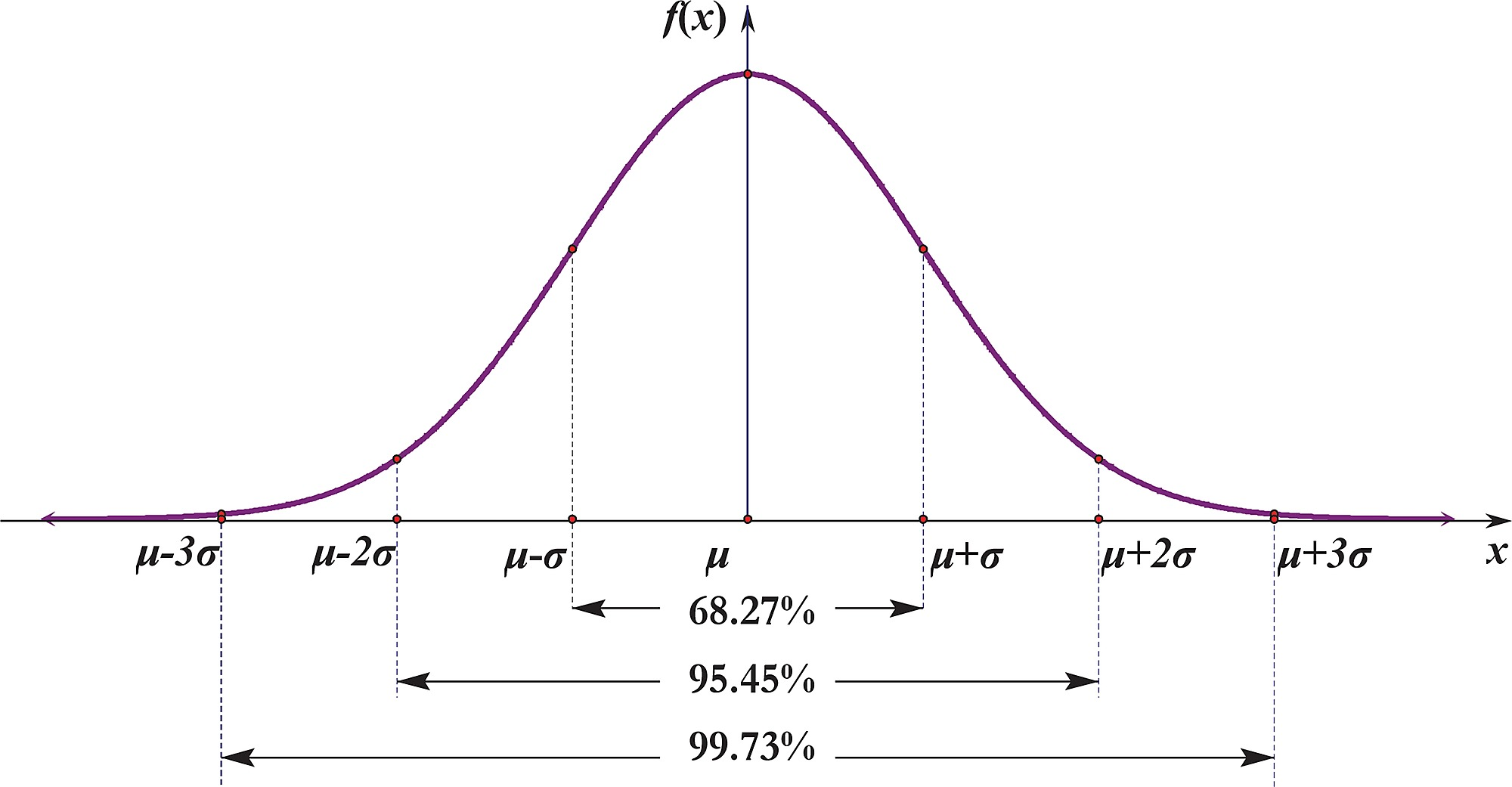# *Geek Box: Using Z-scores in Research

#### *Geek Box: Using Z-scores in Research

To understand and interpret the findings in this study, it will be helpful to get to grips with standard scores, known in research as “z-scores”. Calculating z-scores is based on the normal distribution of data, as shown in the figure below.A normal distribution appears as a bell-curve shape; ignore the Latin text, and just look at the lines and numbers. Dead centre we have the mean; this could be average height, weight, or blood pressure, in the population. Then the dashed lines either side of the mean are the standard deviation [SD] from the mean, i.e., what proportion of the data varies from the mean, and by how much. In a normal distribution, the variance is equal either side – above and below – the mean. Thus, you can see that 68.27% is within 1-SD from the mean; 95.45% within 2-SD; and 99.73% within 3-SD.

A z-score takes a set of data and standardises it to a normal distribution. Let’s bring this to life with an example relevant to the present study. Assume we are interested in a cognitive test that assesses memory by testing the ability of participants to recall up to 10 words. Thus, the possible scores range from 0 to 10. Let’s say the mean score in the study was 5, and the SD was 3.

One participant has scored 6; we might intuitively think that 6/10 is a good score, but how do we know how it compares to the overall study group? This is where researchers will calculate a z-score. The participant’s z-score is calculated as; 6 [the score] minus 5 [the mean], divided by 3 [the SD]: 0.33. By calculating a z-score for every participant, it is then possible to use these scores to compare how well that participant did compared to others.

If another cognitive test was used that scored on a different scale, it would also be possible to calculate z-scores for that test to then compare how participants scored on both tests.